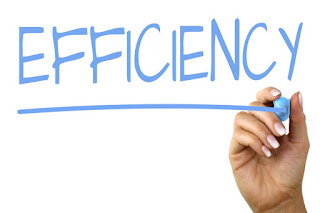# Efficiency

## Efficiency

• The efficiency of a device or of a circuit a circuit in the ratio of useful output to the input.

I.e.     Efficiency = useful output / input

• The law of conservation of energy states that think "energy cannot be created or can't be destroyed but it can be converted from one form to another form".Efficiency

• Some of the energy in electric circuit may be converted into a form that is not useful for example consider an electric motor the purpose of motor is to convert electrical energy into mechanical energy it does this but it also converts a part of input energy into heat. the heat produced is not useful therefore the useful output energy is less than the input energy in other words the efficiency of the motor is less than 100%.

• Some electrical devices are nearly nearly 100% efficient an electric heater is an example in a heater practically all the input electrical energy is converted into heat energy in this case the heat is useful output